normal distribution

(redirected from Gauss distribution)
Also found in: Dictionary, Thesaurus, Medical, Financial.
Related to Gauss distribution: Gaussian random variable

normal distribution

[′nȯr·məl ‚di·strə′byü·shən]
(statistics)
A commonly occurring probability distribution that has the form where e is the mean and σ is the variance. Also known as Gauss' error curve; Gaussian distribution.
McGraw-Hill Dictionary of Scientific & Technical Terms, 6E, Copyright © 2003 by The McGraw-Hill Companies, Inc.

normal distribution

a continuous distribution of a random VARIABLE with its mean, median and mode equal (see MEASURES OF CENTRAL TENDENCY). Thus the normal curve is symmetrical, and bell-shaped as in Fig. 21 below. See also PROBABILITY, PARAMETRIC STATISTICS assume the parent population to have a normal distribution. In reality, a normal distribution is only approximated, and this is regarded as acceptable to fulfil this requirement of a parametric test.
Collins Dictionary of Sociology, 3rd ed. © HarperCollins Publishers 2000
The following article is from The Great Soviet Encyclopedia (1979). It might be outdated or ideologically biased.

Normal Distribution

one of the most important probability distributions. The term “normal distribution” refers both to probability distributions of random variables and to joint probability distributions of several random variables, that is, to distributions of random vectors.

The probability distribution of a random variable X is said to be normal if it has a probability density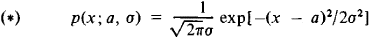The family of normal distributions (*) thus depends on the two parameters a and σ. Here, the expected value of X is equal to a and the variance of X is equal to σ2. The graph of the normal density Y= p(x; a,σ) is symmetric with respect to the ordinate, which passes through the point x = a, and has at this point a unique maximum equal to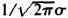. The normal density curve becomes increasingly narrow as σ decreases (see Figure 1). Changing a with σ held constant does not alter the form of the curve but causes it to be displaced along the abscissa. The area under the normal curve is always equal to unity. When a = 0 and σ = 1, the corresponding distribution function is equal to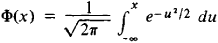In the general case, the normal distribution function F(x; a,σ) can be calculated by the formula F (x; a, σ) = Φ (t), where t = (x — a)/σ. Extensive tables have been compiled for the function Φ(t) and for several derivatives of the function. For a normal distribution, the probability that the inequality ǀX — a ǀ > kσ will be satisfied, which is equal to 1 — Φ(k) + Φ(–k), decreases extremely rapidly as k increases (see Table 1). The possibility of deviations from a that exceed 3σ is therefore disregarded in many practical problems involving a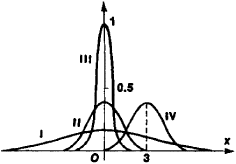Figure 1. Normal-distribution density curves for various values of the parameters a and σ : (I) a = 0, σ = 2.5; (II) a = 0, σ = 1; (III) a = 0, σ = 0.4; (IV) a = 3, σ = 1

normal distribution—the three-σ rule. (As can be seen from Table 1, the corresponding probability is less than 0.003.) The probable deviation for the normal distribution is 0.67449σ

Table 1
kProbability
1 .....................................0.31731
2 .....................................0.04550
3 .....................................0.00269
4 .....................................0.00006

Normal distributions are encountered in a large number of applications. Attempts to explain this have long been made. Theoretical justification of the exceptional role of the normal distribution is given by the limit theorems in probability. The relevant result can be qualitatively explained in the following manner. The normal distribution serves as a good approximation whenever the random variable under consideration represents the sum of a large number of independent random variables, with the largest variable being small in comparison with the sum.

The normal distribution may also appear as an exact solution for some problems—within the framework of the mathematical model that is used for the problem. This is the case in the theory of random processes (in one of the principal models of Brownian motion). Classical examples of the normal distribution as an exact distribution were given by K. Gauss (the distribution of errors of observations) and J. Maxwell (the distribution of molecular velocities).

The joint distribution of several random variables X1, X2, …, Xs is said to be a multivariate normal distribution if the corresponding probability density has the form

p(x1, …, xn) = C exp[–Q(x1 - a1, …, xs - as)]

where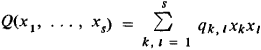in which qk, l = ql, k is a positive definite quadratic form. The constant C is determined from the condition that the integral of p over all space be equal to unity. The parameters a1, …, as are equal to the expected values of X1, …, Xs, respectively, and the coefficient qk, l can be expressed in terms of the variances σ21,: . ., σ2s of these variables and the correlation coefficient pk, l for Xk and Xi. The total number of parameters defining a normal distribution is equal to

(s + 1)(s + 2)/2 - 1

and increases rapidly with s; the number of parameters equals 2, 20, and 65, respectively, when s =1,5, and 10. The multivariate normal distribution is the basic model for multidimensional statistical analysis. It is also used in the theory of random processes, where the normal distribution in infinite-dimensional spaces is also considered.

For information on problems associated with the estimation of normal-distribution parameters on the basis of observational results, seeSMALL SAMPLES and UNBIASED ESTIMATE. For verification of the assumption of normality, seeNONPARAMETRIC METHODS (in mathematical statistics).

IU. V. PROKHOROV

normal distribution

(statistics)
(Or "Gaussian distribution") The frequency distribution of many natural phenomena such as the height or intelligence of people of a certain age and sex. The formula looks something like:

P(x) = e^(((x-m)/s)^2)

where P(x) is the probability of a measurement x, m is the mean value of x and s is the standard deviation.

Also known as a "bell curve" because of its shape.